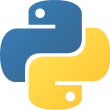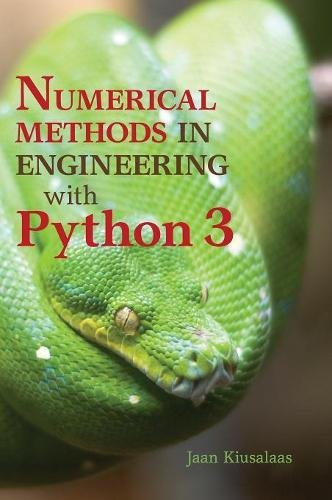Language
• Python 3Reading time
• Approximately 43 daysWhat you will learn
• EngineeringAuthor
• Jaan KiusalaasPublished
• 10 years, 10 months agoPackages you will be introduced to
• numpy
• matplotlibThis book is an introduction to numerical methods for students in engineering. It covers the usual topics found in an engineering course: solution of equations, interpolation and data fitting, solution of differential equations, eigenvalue problems, and optimization. The algorithms are implemented in Python 3, a high-level programming language that rivals MATLAB® in readability and ease of use. All methods include programs showing how the computer code is utilized in the solution of problems. The book is based on Numerical Methods in Engineering with Python, which used Python 2. This new text demonstrates the use of Python 3 and includes an introduction to the Python plotting package Matplotlib. This comprehensive book is enhanced by the addition of numerous examples and problems throughout.
The author Jaan Kiusalaas has the following credentials.

• Works/Worked at NASA